## Indicators

•Cited by SciELO
•Access statistics

•Cited by Google
•Similars in SciELO
•Similars in Google

## Print version ISSN 0012-7353

### Dyna rev.fac.nac.minas vol.85 no.204 Medellín Jan./Mar. 2018

#### http://dx.doi.org/10.15446/dyna.v85n204.67589

Artículos

Analysis of pressure and pressure derivative interference tests under linear and spherical flow conditions

Análisis de presión y derivada de presión en pruebas de interferencia en condiciones de flujos lineal y esférico

Freddy Humberto Escobar-Macualoa  , Esteban Rojas-Borregoa  , Neila Tatiana Alarcón-Olayaa

a Grupo de Investigación GIPE de la Facultad de Ingeniería, Universidad Surcolombiana, Neiva, Colombia. fescobar@usco.edu.co, rojasborrego@gmail.com, ntalarcon123@gmail.com

Abstract

Often, interpretation of interference tests is performed for systems acting under radial flow regimen conditions by means of conventional straight-line method, type-curve matching and TDS technique. For linear and spherical flow cases, the interpretation of interference tests is performed by the conventional analysis and type-curve matching. These procedures do not allow verification of the estimated parameters; therefore, this paper presents the formulation of a more practical, useful and accurate methodology which is achieved based upon the determination of characteristic features found on the pressure and pressure derivative versus time log-log plot with the purpose of developing analytical expressions for the interpretation of interference tests under spherical and linear flow conditions. These equations were successfully verified by their application on synthetic tests.

Key words: interference testing; linear flow; spherical flow; steady state; pseudosteady state

Resumen

Normalmente, la interpretación de las pruebas de interferencia se realiza en sistemas que actúan bajo condiciones de régimen flujo radial utilizando análisis convencional de la línea recta, curvas tipo y técnica TDS. Para los casos de flujos lineal y esférico, la interpretación de pruebas de interferencia se realiza mediante el método convencional y el ajuste con curvas tipo. Estos procedimientos no permiten verificación de los parámetros estimados, y por ello en este trabajo se presenta una formulación de una metodología más práctica, útil y práctica mediante el uso de rasgos características en el gráfico logarítmico de la presión y derivada de presión contra tiempo, que permitan desarrollar expresiones analíticas directas usadas para la caracterización del yacimiento en pruebas interferencia en condiciones de flujos esférico y lineal. Estas ecuaciones se verificaron satisfactoriamente mediante a su aplicación a pruebas sintéticas.

Palabras-clave: pruebas de interferencia; flujo lineal; flujo esférico; estado estable; estado pseudoestable

1. Introducción

Normally, the methods used for interference tests interpretation are type-curve matching and the conventional straight-line method. In the study by  on water flow in aquifers and its influence on water-producing wells, he took the first steps in developing a graphical analysis method that intermediate the field data and the theoretical data for radial regime, which is known as type-curve matching method. Later [2,12,15,18], among others, improved this form of analysis for interference tests by involving either conventional analysis or the pressure derivative function. Type-curve matching is not only tedious but highly inaccurate with a slight data point reading variation.

The first application of the pressure derivative in interference tests was done by . This method did not have much impact at first because it made use of the arithmetic derivative and secondly the noise introduced to the pressure data by external sources increased with the derivative and before the 90s there was not many studies with the estimate of the pressure derivative function. A subsequent analysis was performed by , taking as reference the work done by  for two-rate testing.

 presented the analytical models for radial, linear and spherical flow regimes and used type-curve matching and regression analysis for the interpretation of interference tests. In addition to presenting the analytical interference solution for radial flow regime,  also presented the solutions for linear and spherical flow regimes. The first one occurs in elongated deposits caused by channeling or faulting and the second one in very thick formations.  followed the philosophy of the TDS Technique, , for interference testing using the intersection between pressure and pressure derivative. Later, these recently mentioned works were applied by  to determine heterogeneities from interference testing. TDS Technique has many applications, just to name a few of them,  and  extended this methodology for interpreting pressure tests in elongated systems, and  developed the TDS for heavy oil obeying power-law behavior. Much more applications of the TDS Technique were compiled by .

Formulating a more practical interpretation methodology for interpretation of interference tests under linear and spherical conditions is the purpose of this paper. For this, the starting points are the linear and spherical solutions presented by  so the behavior of pressure and the pressure derivative curves are generated, and, from observations at characteristic points analytical expressions are developed to allow interpreting interference tests in a simple, practical and accurate way. Additionally, based on the work of [3,10], the presence of either pseudosteady-state or steady-state periods was used to develop expressions for the determination of the well drainage area when the duration of the test allows it. The expressions developed were verified satisfactorily with their application to synthetic tests.

2. Mathematical model

For the development of the TDS Technique, , in interference tests where either linear or spherical conditions are presented, it is necessary to understand the pressure and pressure derivative behavior in each system with the purpose of finding special features or straight lines which allow obtaining mathematical expressions for reservoir characterization.

2.1. Lineal flow regime model

 presented the solution for the pressure distribution in linear systems. This solution was considered for the case of a well producing a constant flow and infinite system. In addition, for an interference test, it was assumed that only half of the active well flow rate is perceived in the observer well. The pressure drop for the described condition is:

And the pressure derivative is given by:

The dimensionless parameters are given as:

Eqs. (1) and (2) allowed generating several pressure and pressure curves as given in Fig. 1 using data from Table 1. As either the reservoir width, b, or the distance between the wells, L, or the reservoir thickness, h, varies so do the pressure and pressure derivative curves; therefore, a unique behavior was obtained after multiplying both dimensionless pressure and pressure derivative and dimensionless time values by certain parameters as shown in Fig. 2. Notice in Eq. (3) that the squared wellbore radius can be changed by drainage area obtained the dimensionless time based on area, tDA .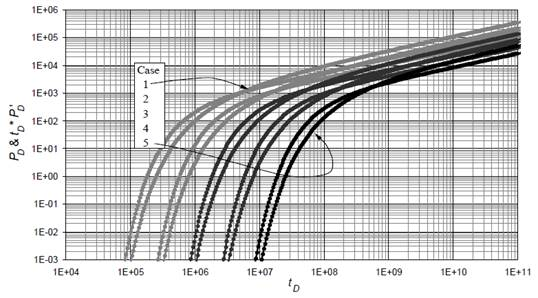Source: The authors

Figure 1 Dimensionless pressure and pressure derivative behavior for interference tests under linear flow conditions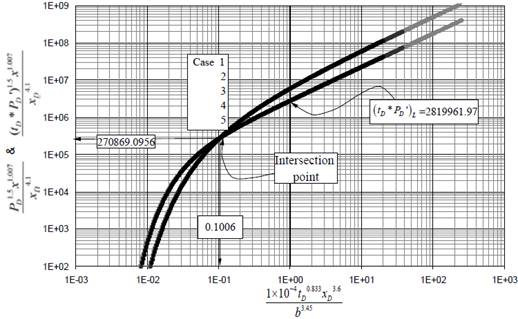Source: The authors

Figure 2 Unified pressure derivative behavior interference tests under linear flow conditions

From the unified behavior observed in Fig. 2, a unique intersection point with coordinates 270869.0956, 0.1006 is found:

After replacing the dimensionless time and the definition of dimensionless length, xD =x/L, in Eq. (6), an expression to find porosity was obtained:

The abscise value was:

From which permeability is solved for once Eq. (4) and xD , are replaced in the above expression:

Since the pressure and pressure derivative values are the same, Eq. (9) can also use the pressure derivative value, instead.

The governing equation for the unique linear flow regime was obtained by regression analysis to be:

Replacing the dimensionless parameters in Eq. (10) is possible to obtain an expression to find permeability using an arbitrary point during linear flow regime:

Because of the noise introduced to the pressure readings from external sources, it is recommended to read the pressure derivative value during linear flow at a time, t = 1 hr, to obtain a more representative reading value, then, Equation (11) becomes:

The dimensionless pressure governing was found by integration of Eq. (10); therefore:

Dividing Eq. (13) by Eq. (10), and solving for the linear skin factor and replacing the dimensionless quantities, it yields:

2.2. Spherical flow regime model

The governing pressure drop equation for a well producing a constant flow was given by  when spherical flow is developed in a reservoir is given by:

Its pressure derivative is then:

As for the former case, pressure and pressure derivative curves were generated, for this case with data from Table 2, considering the variation of wellbore radius, rw , reservoir thickness, h, and distance between wells, r. Such curves are reported in Fig. 3.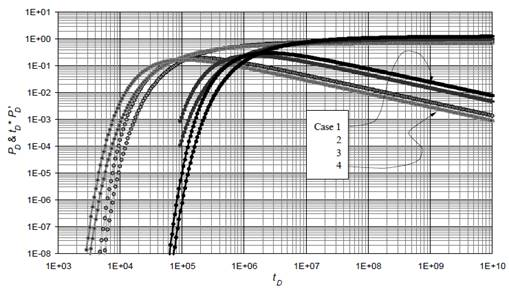Source: The authors

Figure 3 Dimensionless pressure and pressure derivative behavior for interference tests under spherical flow conditions

It was also necessary to unify the pressure derivative curves to obtain a universal behavior. It was performed by multiplying both dimensionless pressure, pressure derivative and time for certain factors as shown in Fig. 4.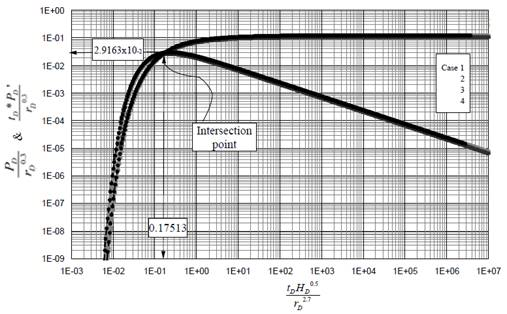Source: The authors

Figure 4 Unified pressure derivative behavior interference tests under spherical flow conditions

From the unified behavior observed in Fig. 4 a unique intersection point with coordinates 0.029163, 0.17513 is found. Therefore,

From which porosity is obtained once the dimensionless quantities are used:

and;

After replacing Eq. (4), the definition of dimensionless radius and solving for the formation permeability, it yields.

Since the pressure and pressure derivative values are the same, Eq. (20) can use the pressure derivative value, instead.

The governing universal equation for spherical flow was obtained by linear regression to be:

Replacing the dimensionless parameters in Eq. (21), it is possible to obtain an expression to find permeability using an arbitrary point during linear flow regime:

As for the linear case, because of the noise, it is recommended to apply Eq. (22) at the time of 1 hr.

The dimensionless pressure governing equation was obtained by integration of Eq. (25), to be:

Dividing Eq. (23) by Eq. (21), replacing the dimensionless quantities and solving for the spherical skin factor will result:

2.3. Late time behavior

The basis for interference in bounded systems were given by . The application of the pressure derivative for limited reservoirs was presented by . This means that it is feasible to develop either pseudosteady-state or steady-state periods during an interference test. As observed in Fig. 5, once spherical flow regime vanishes pseudosteady-state period obeys the following governing pressure derivative equation:

Replacing the dimensionless quantities and solving for the drainage area, it results:

As shown in Fig. 6 the pressure derivative governing equation is given by:

As for the spherical flow case, after replacing the dimensionless time based on area, the resulting area equation was: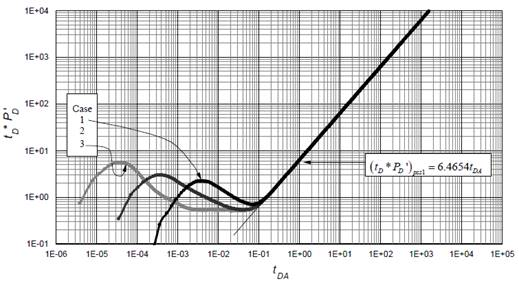Source: The authors

Figure 5 Dimensionless pressure behavior versus dimensionless time based on area for spherical flow interference in closed systems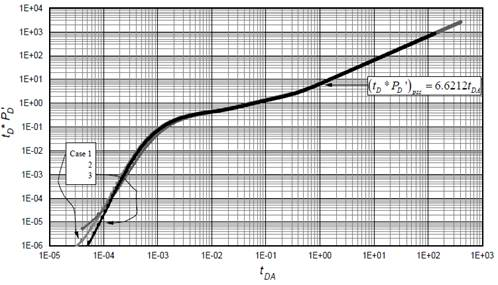Source: The authors

Figure 6 Dimensionless pressure behavior versus dimensionless time based on area for linear flow interference in closed systems

On the other hand, for constant-pressure bounded systems, Figs. 7 and 8, the late-time governing pressure derivative equations for linear and spherical flow regimes, respectively are given by:

Replacing the dimensionless quantities and solving for the drainage area results in:

As shown in Fig. 8 the pressure derivative governing equation is given by:

Replacing the dimensionless quantities and solving for the drainage area, it results: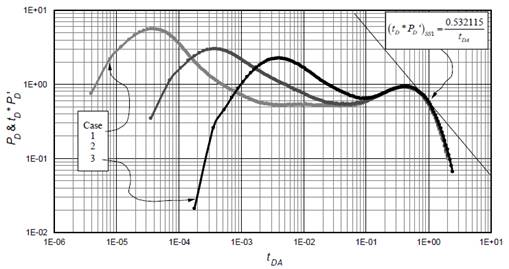Source: The authors

Figure 7 Dimensionless pressure behavior versus dimensionless time based on area for spherical flow interference in constant-pressure systems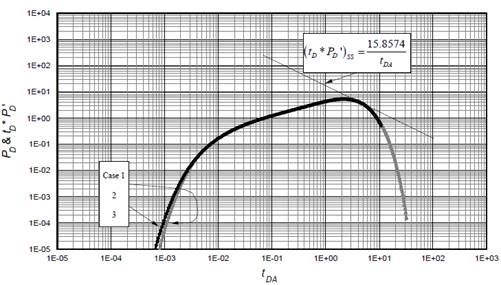Source: The authors

Figure 8 Dimensionless pressure behavior versus dimensionless time based on area for linear flow interference in constant-pressure systems

Because of the noise, it is recommended to apply Eqs. (28), (30) and (32) at time of 1 hr.

The spherical system can be readily converted to hemispherical system. As seen in Eq. (15), the constant (sp has the value of 70.6. For hemispherical flow conditions this constant is multiplied by two, taking the value of 141.2, so do the constants in the related equations. Apart from this, appendix A presents the gas flow equations for linear and spherical flow conditions.

3. Examples

Two synthetic examples were generated to validate the porosity and permeability equations and two other tests were synthetically created two verify the equations of area. Table 3 contains the input data for the examples.

3.1. Example 1 (Linear)

The simulated data provided in Fig. 9 was generated with data from Table 3, from where the following information was read: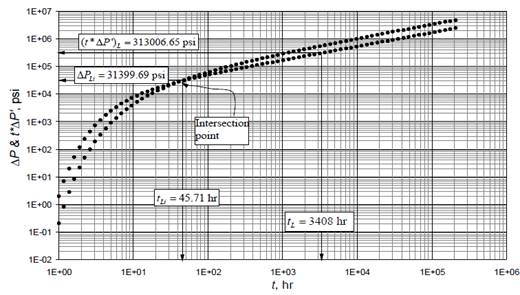Source: The authors

Figure 9 Log-log of pressure and pressure derivative versus time for example 1 (linear case)

Then, permeability was estimated with Eqs. (9) and (11) and porosity with Eq. (7). Results are reported in Table 4.

3.2. Example 2 (spherical)

Fig. 10 presents the pressure and pressure derivative data for a synthetic test under spherical flow interference conditions which used input data from Table 3. The following information was read from such plot: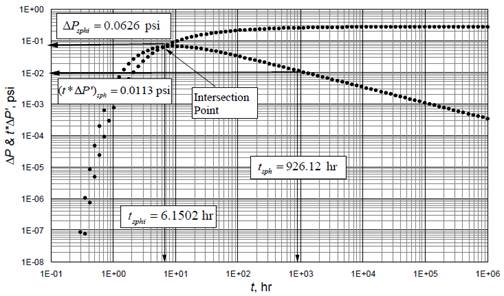Source: The authors

Figure 10 Log-log of pressure and pressure derivative versus time for example 2 (spherical case)

Permeability was estimated using Eqs. (20) and (22). Porosity was found with Eq. (18). Results are also reported in Table 4.

3.3. Example 3 (spherical case)

A long pressure test was also simulated with input data from Table 3. The pressure and pressure derivative for this example is reported in Fig. 11 from where the following characteristic point was read:

Drainage area was estimated with Eq. (30) -reading at t = 1 hr- and reported in Table 4.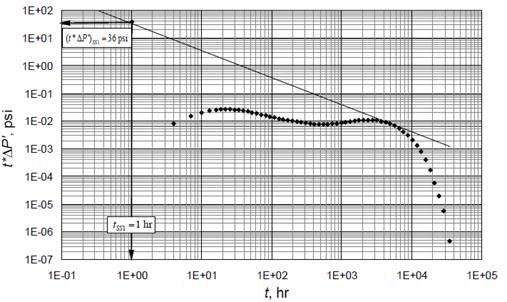Source: The authors

Figure 11 Log-log of pressure and pressure derivative versus time for example 3 (spherical case)

3.4. Example 4 (linear case)

The pressure and pressure derivative versus time data reported in Fig. 12 was also obtained using data from Table 3. The following datum was read from this figure.

Drainage area was estimated with Eq. (28) -reading at t = 1 hr- and also reported in Table 4.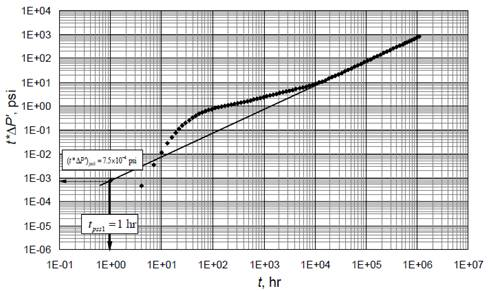Source: The authors

Figure 12 Log-log of pressure and pressure derivative versus time for example 4 (linear case)

4. Conclusions

1. The intersection point, a characteristic one-half slope (for linear flow) and negative one-half slope (for spherical) flow, a late time unit-slope line (pseudosteady state) and a late time negative unit-slope line (steady state) are identified and set as characteristic features on the pressure and pressure derivative versus time log-log plot which allow developing expressions for reservoir characterization.

2. TDS Technique is applied to interference testing under linear and spherical flow regime conditions. A unique point of intersection - found for each case - is used to find expressions to find reservoir permeability and porosity. Also, the permeability value is verified by another expression that uses an arbitrary point read on each flow regime (linear or spherical). There are also developed equations to estimate the drainage area in both dealt flow regimes for close or constant-pressure boundary systems. The Equations are successfully verified on synthetic cases.

Nomenclature

Suffixes

Greek

References

 Agarwal, R.G., Real gas pseudo-time - A new function for pressure buildup analysis of MHF gas wells. Society of Petroleum Engineers. January 1. 1979, DOI: 10.2118/8279-MS. [ Links ]

 Al-Marhoun, M.A., Interference testing: A new analysis approach. Society of Petroleum Engineers, 1985. DOI: 10.2118/13732-MS. [ Links ]

 Brown, M.W. and Ambastha, A.K., Interference test analysis for rectangular reservoirs. Petroleum Society of Canada. January 1. 1991, DOI: 10.2118/91-45. [ Links ]

 Chatas, A.T. Unsteady spherical flow in petroleum reservoir. SPE J. 6(2), pp. 102-114; 1966. Trans., AIME, 237. SPE-1305-PA. DOI: 10.2118/1305-PA. [ Links ]

 El-Khatib, N.A.F., A new approach to interference test. SPE Formation Evaluation, 2(4): pp. 609-610. 1987. Society of Petroleum Engineers. DOI: 10.2118/13733-MS. [ Links ]

 Escobar, F.H., Hernández, Y.A. and Hernández, C.M., Pressure transient analysis for long homogeneous reservoirs using TDS technique. Journal of Petroleum Science and Engineering. 58(1-2), pp. 68-82, 2007. DOI: 10.1016/j.petrol.2006.11.010. [ Links ]

 Escobar, F.H., Cubillos, J., Montealegre-M., M., Estimation of horizontal reservoir anisotropy without type-curve matching. Journal of Petroleum Science and Engineering 60, pp. 31-38. 2008. DOI: 10.1016/j.petrol.2007.05.003. [ Links ]

 Escobar, F.H., Montealegre-M., M. and Carrillo-Moreno, D., Pressure and pressure derivative transient analysis without type-curve matching for elongated reservoirs with changes in permeability or width. CT&F - Ciencia, Tecnología y Futuro. 4(1), pp. 75-88, 2010. [ Links ]

 Escobar, F.H. Recent advances in practical applied well test analysis. Nova publishers, New York. Published by Nova Science Publishers, Inc. † New York, 2015. [ Links ]

 Foster, G.A., Wong, D.W., Asgarpour, S. and Cinco-Ley, H., Interference test analysis in limited reservoirs using the pressure derivative approach: Field example. Petroleum Society of Canada. January 1, 1994. DOI: 10.2118/94-30. [ Links ]

 Jacob, C.E., On the flow of water in an elastic artesian aquifer. American Geophysical Union 21, pp. 574-588. 1940. DOI: 10.1029/TR021i002p00574. [ Links ]

 Kamal, M.M., Interference and pulse testing-A review. Journal of Petroleum Technology, Society of Petroleum Engineers. 35(12), pp. 2257-2270, 1983. DOI: 10.2118/10042-PA. [ Links ]

 Martinez, N.R. and Samaniego, F.V., Advances in the analysis of pressure interference tests. Journal of Canadian Petroleum Technology Society of Petroleum Engineers, 49(12), pp. 65-70, 2010. DOI: 10.2118/141028-PA. [ Links ]

 Martinez, J.A., Escobar, F.H. and Cantillo, J.H., Application of the TDS technique to dilatant Non-Newtonian/Newtonian Fluid composite reservoirs. Ingeniería e Investigación Journal. 31(3), pp. 130-134, 2011. DOI: 10.15446/ing.investig [ Links ]

 Matthies, E.P., Practical application of interference tests. Journal of Petroleum Technology, Society of Petroleum Engineers , 16(03), pp. 1-4, 1964. DOI: 10.2118/627-PA. [ Links ]

 Miller, F.G., Theory of unsteady-State influx of water in linear reservoirs. Journal Institute of Petroleum 48(467), pp. 365-379, 1962. [ Links ]

 Ouandlous, A., Interpretation of interference test by Tiab’s direct synthesis technique, MSc. Thesis. University of Oklahoma, United States, 1999. [ Links ]

 Tiab, D. and Kumar, A., Application of the P’D function to interference analysis. Society of Petroleum Engineers, 1980. DOI: 10.2118/6053-PA. [ Links ]

 Tiab, D., Analysis of pressure and pressure derivative without type-curve matching: 1- Skin and wellbore storage, Journal of Petroleum Science and Engineering , 12(3), pp. 171-181, 1995. DOI: 10.1016/0920-4105(94)00040-B. [ Links ]

How to cite: Escobar-Macualo, F.H., Rojas-Borrego, E. and Alarcón-Olaya, N.T., Analysis of pressure and pressure derivative interference tests under linear and spherical flow conditions. DYNA, 85(204), pp. 44-52, March, 2018.

Appendix A. Gas flow Equations

 introduced the pseudotime function to account for the time dependence of both gas viscosity and total system compressibility:

This function is better defined as a pressure function given in hr psi/cp:

Now, μ and ct are pressure dependent properties. Rewriting Eq. (3) can be rewritten as:

Including the pseudotime function, ta (P), in Eq. (A.3), the dimensionless pseudotime is given by:

Multiplying and, then, dividing by ( μct ) i a similar equation to the general dimensionless time expression;

The dimensionless pseudopressure and pseudopressure derivative are defined by:

For gas linear flow regime interference, Eqs. (7), (9) and (12) become:

For spherical gas flow regime interference, Eqs. (18), (20) and (22) become:

For late time behavior, Eqs. (26), (28), (30), and (32) become:

Received: September 08, 2017; Revised: October 30, 2017; Accepted: November 11, 2017This is an open-access article distributed under the terms of the Creative Commons Attribution License